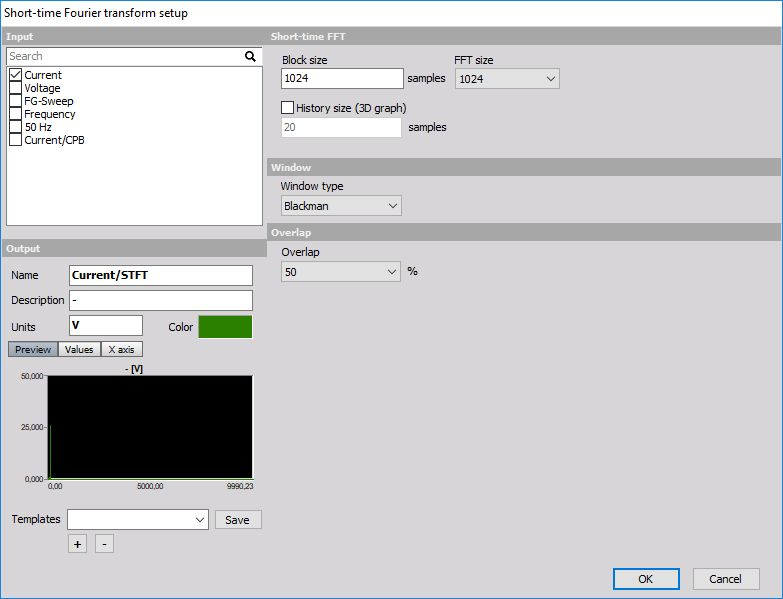# Short-time Fourier transform

Short time Fourier transformation is the procedure which calculates more lines than the normal FFT. This is achieved by having a smaller real block size of data and larger FFT size. Real data is windowed and zero padded and then FFT is calculated. With this procedure, we can calculate more FFT’s for the same time base. It is nice for use with fast transients.• Block size - defines the number of real data samples to be taken for the calculating FFT.
• FFT size - defines the number of resulting lines and with that the ratio between real and zero padded lines.
• Window type - describes the FFT window to be used. There is a good description of the usage of different window functions in the tutorial. By default, we use Blackman, because it is a good compromise between the amplitude error and width of sidebands.
• Overlap - defines how much will two FFT shots overlap between each other. 50% is enough that all the samples will have the same weight as the result independent of the window which is used.

STFT as can be viewed in 2D and 3D graph.